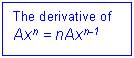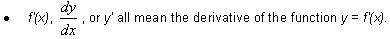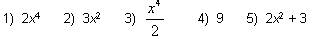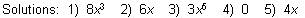# Introduction to Differentiation Math Review

math tutorials > introduction to differentiation

## What is differentiation? Definition of a derivative.

Differentiation is the process of finding the derivative f'(x) of a function f.  It is a part of maths called Calculus

The derivative is the gradient of the tangent of the graph of f at the point x.## Differentiation examples

 f(x) = x³ f'(x) = 3x² f(x) = 2x² f'(x) = 2 x 2x = 4x f(x) = 7x³ f'(x) = 7 x 3x² = 21x²

When an expression has several terms, find the derivative of each term separately.  You may need to expand brackets first.

 f(x) = 3x³ + 2x² f'(x) = 9x² + 4x

## Note:

• The derivative of x is 1.
• The derivative of any number (e.g. 1) is 0.## Differentiation problems for you to try.

Differentiate: